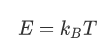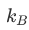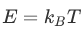###Dimensional Analysis

Energy

In our previous discussions of kinematics(the study of the motion of particles) we only encountered two fundamentalphysical quantities: space and time. However, if we want to talk about theenergy of a particle, we also need to consider the amount of matter that ismoving, i.e. the mass of the particle (m). We will call M the dimension of thisthird fundamental quantity:

[m]=M

The dimensions of all the physicsquantities you will ever encounter in mechanics can be described in terms of L,T, M.

The Boltzmann Constant

Thermodynamics and the molecular theory ofheat have their own fundamental physical quantity - temperature, T. However,because we have used T to refer to the dimension of time, we will use the Greekletter "theta", Θ, to refer to the dimensions of temperature:

热力学和热分子理论有自己的基本的物理量-温度，但是，因为我们已经用T指时间维度，我们将使用希腊字母“θ”，参考温度的维度：

[T]=Θ

Caution: In the case of the quantity oftime t we have defined its dimension to be T, and we wrote: [t]=T. This iswhere the bracket notation comes in handy because it keeps a clear distinctionbetween the quantities and the dimensions.

The success of the molecular theory of heatwas based on its ability of relating microscopic (connected to the motion ofmolecules) and macroscopic variables (describing the whole system of particlessuch as temperature or pressure). One such expression that bridged the microscopicand macroscopic was:Here one relates the kinetic energy ofmolecules (depending only on their mass and velocity) to the temperature of thegas - i.e. the whole system of molecules. (This is not quite the preciseexpression of the energy. There are a few extra dimensionless factors that needto be included to account for the different types of motion that a molecule canhave. For simplicity, we have dropped the extra numerical factors. For thepurpose of this course, this will not make a difference.)is called the Boltzmann constant (afterLudwig Boltzmann) and it is a fundamental constant of physics, similar to thespeed of light.被称为玻尔兹曼常数（在路德维希·玻尔兹曼之后），它是物理学的基本常数，类似于光的速度。

The Planck Constant

In the lesson about the PhotoelectricEffect we have encountered another formula for energy:

E=hv

where v is the frequency of a wave, and Ethe energy of the wave. h is the Planck constant, also a fundamental constantof physics.

The meaning of this formula is very similartoas it relates a microscopic and a macroscopic description of the samephenomena. If in the case of the Brownian Effect, the temperature is themacroscopic quantity characterizing the system, in the case of thePhotoelectric Effect, the frequency of the waves are the equivalent macroscopicquantity. As in the case of temperature, the wave frequency is an observablequantity and it can easily be measured.

In both cases E refers to the kineticenergy of some unobservable (at the time) particles: molecules in the case ofthe Brownian Effect, and photons in the case of the Photoelectric Effect.

If we also include Einstein's famousrelation between energy and mass, we have three, structurally similar formulas:

E=mc2 (Einstein)

E=hν (Planck)

E=kBT (Boltzmann)

There are subtle differences between theseexpressions, and the way he have interpreted them has changed throughout time.It is enough to remember that in his Photoelectric Effect Paper, Einsteinborrowed Planck's equation and applied it to light - an unintended applicationfor Planck这些表达方式之间有细微的差别，他解释它们的方式在整个时间里都发生了变化。记住，在他的光电效果文件中，爱因斯坦借用了普朗克的公式并应用于光这是普朗克的一个意外应用。             .

In all three cases one connects an energyto a macroscopic quantity (mass, frequency or temperature) using a fundamentalconstant. These are not ad-hoc or empirical formulas, but they express afundamental physical connection.